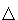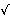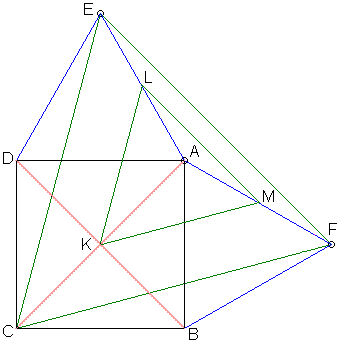Thébault's Problem II: What Is It? A Mathematical Droodle

ExplanationThébault's Problem II

The applet suggests the following theorem (Victor Thébault, 1937):

On the sides AB and AD of the square ABCD erect equilateral triangles AED and AFB -- both either on the inside or the outside of the square. Then triangle CEF is also equilateral.Proof 1

Consider, for example, the case where E and F are located outside the square ABCD. First of all, triangles CDE and and CBF are equal. Indeed, CD = CB and DE = BF. Also, ∠CDE = 90° + 60° = ∠CBF.

Next, ∠DCE + ∠BCF = ∠DCE + ∠DEC = 180° - 150° = 30°. Therefore, inCEF, ∠ECF = 90° - 30° = 60° and also CE = CF.CEF is thus equilateral.

Proof 2

Let's make a use of complex variables. Assume the origin is at C and let u and v be two complex numbers corresponding to B and D, respectively. In fact, u = ir, v = r, for some positive real number r. Let a be the counterclockwise rotation through 60°. 1/a = a-1 then is the rotation through -60°. a =3/2 + i/2. a-1 = 3/2 - i/2.

E corresponds to the complex number u + av, F corresponds to v + u/a = (av + u)/a. The ratio of the two numbers is a, which exactly means that the length of CE and CF are equal (this is because |a| = 1), while the angle between the two is 60°. This completes the proof. But we can easily establish an additional fact.

The area of the equilateral triangle of side x equals 3·x²/4.

We just found that the side of the equilateral triangle CEF that was constructed outwardly equals u + av. For the one constructed inwardly the side is given by u + v/a. Omitting the coefficient3/4, the sum of areas of the "outward" and the "inward" triangles CEF is |u + av|² + |u + v/a|².

Since |a| = |1/a| = 1, we obtain

 |u + av|² + |u + v/a|² = |u + av|² + |au + v|² = |u|² + |av|² + |au|² + |v|² = 2(|u|² + |v|²),

which means that the total area of two triangles CEF (one constructed outwardly, the other inwardly) equals twice the combined area of triangles ABF and ADE.

Proof 3Let K be the point of intersection of the diagonals AC and BD, L and M the midpoints of AE and AF. Consider ΔKLM. Its vertex K is midway between vertices D and B of triangles AED and AFB, vertex L is midway between E and A (in ΔAFB), and vertex M is midway between A (in ΔAED) and F. According to a Three Similar Triangles theorem, ΔKLM is similar to both ΔAED and ΔAFB and, hence, is equilateral. However, triangles KLM and CEF having pairwise parallel sides are similar. Therefore ΔCEF is equilateral.

Proof 4

Proof 3 works for an unexpected generalization of Thébault's problem, which also has independent proofs. Thébault's problem is then obtained as a specific case.Thébault's Problems

• Thébault's Problem I
• Thébault's Problem II
• Thébault's Problem III
• Y. Sawayama's Lemma
• Jack D'Aurizio Proof of Sawayama's Lemma
• Y. Sawayama's Theorem
• Thébault's Problem III, Proof (J.-L. Ayme)
• Circles Tangent to Circumcircle
• Thébault's Problem IV
• A Property of Right Trapezoids
• A Lemma on the Road to Sawayama
• Excircles Variant of Thébault's Problem III
• In the Spirit of Thebault I
• Dao's Variant of Thebault's First Problem
•